One type of math question you will commonly see on the GRE exam is a question related to prime factorization. The following example is similar to the type of question you can expect to see on this topic on the GRE exam:

### Problem:

What is the greatest integral value of k such that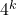is a factor of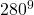?

### Approach:

It is better to work the other way round. Rather than finding the value of k, we prime factorize 280. In fact, finding all the prime factors of 280 is not required. We just need the power of 2, which appears in its factorization.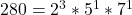So,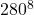will have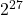as the highest value of 2. This also means that the maximum power of 4 is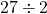which is 13.5. Since 13.5 is not possible, the highest value is 13.

Here’s the video solution: